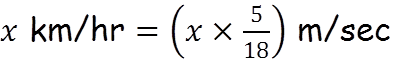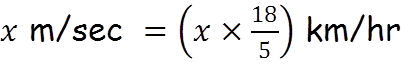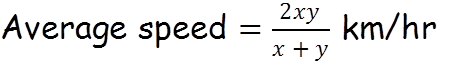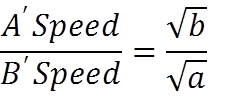# Time & Distance

## Unit Conversion – kmph ⇔ mps## Shortcut Formulas for Time and Distance

Rule 1: If some distance is travelled at x km/hr and the same distance is travelled at y km/hr then the average speed during the whole journey is given byRule 2: If a person travels a certain distance at x km/hr and returns at y km/hr, if the time taken to the whole journey is T hours then the one way distance is given byRule 3: If two persons A and B start their journey at the same time from two points P and Q towards each other and after crossing each other they take a and b hours in reaching Q and P respectively, thenRule 4: If the same distance is covered at two different speeds S1 and S2 and the time taken to cover the distance are T1 and T2, then the distance is given by